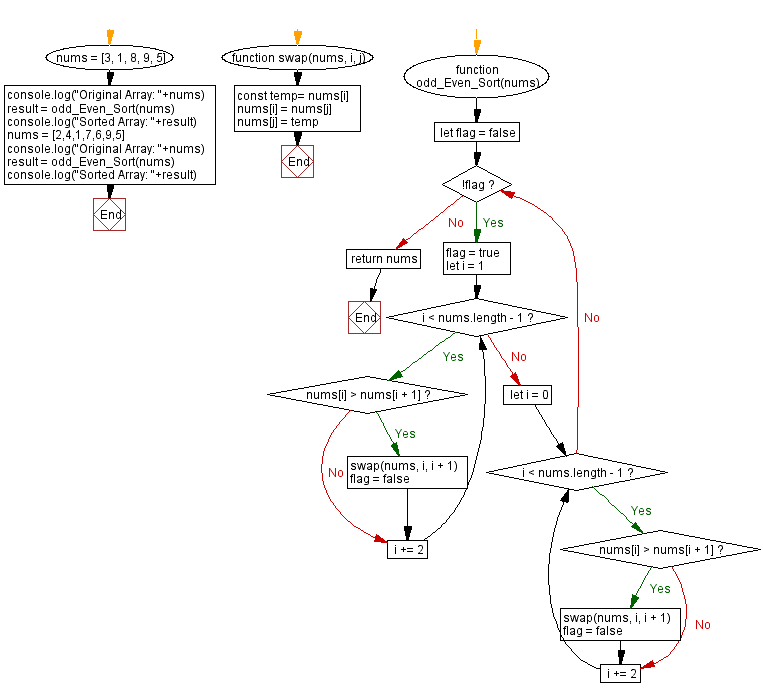# JavaScript Sorting Algorithm: Odd - Even sort

## JavaScript Sorting Algorithm: Exercise-29 with Solution

From Wikipedia,
In computing, an odd–even sort or odd–even transposition sort (also known as brick sort or parity sort) is a relatively simple sorting algorithm, developed originally for use on parallel processors with local interconnections. It is a comparison sort related to bubble sort, with which it shares many characteristics. It functions by comparing all odd/even indexed pairs of adjacent elements in the list and, if a pair is in the wrong order (the first is larger than the second) the elements are switched. The next step repeats this for even/odd indexed pairs (of adjacent elements). Then it alternates between odd/even and even/odd steps until the list is sorted.

Write a JavaScript program to sort a list of elements using the Odd - Even sort algorithm.

Sample Data: Original Array: [3, 1, 8, 9, 5]
Sorted Array: [1, 3, 5, 8, 9]
Original Array: [2, 4, 1, 7, 6, 9, 5]
Sorted Array: [1, 2, 4, 5, 6, 7, 9]

Sample Solution:

JavaScript Code:

``````function swap(nums, i, j) {
const temp= nums[i]
nums[i] = nums[j]
nums[j] = temp
}

function odd_Even_Sort(nums) {
let flag = false
while (!flag) {
flag = true
for (let i = 1; i < nums.length - 1; i += 2) {
if (nums[i] > nums[i + 1]) {
swap(nums, i, i + 1)
flag = false
}
}
for (let i = 0; i < nums.length - 1; i += 2) {
if (nums[i] > nums[i + 1]) {
swap(nums, i, i + 1)
flag = false
}
}
}
return nums
}
nums = [3, 1, 8, 9, 5]
console.log("Original Array: "+nums)
result = odd_Even_Sort(nums)
console.log("Sorted Array: "+result)
nums = [2,4,1,7,6,9,5]
console.log("Original Array: "+nums)
result = odd_Even_Sort(nums)
console.log("Sorted Array: "+result)
```
```

Sample Output:

```"Original Array: 3,1,8,9,5"
"Sorted Array: 1,3,5,8,9"
"Original Array: 2,4,1,7,6,9,5"
"Sorted Array: 1,2,4,5,6,7,9"
```

Flowchart:Live Demo:

See the Pen searching-and-sorting-algorithm-exercise-29 by w3resource (@w3resource) on CodePen.

Improve this sample solution and post your code through Disqus

Previous: Cycle sort.
Next: Pigeonhole sort.

What is the difficulty level of this exercise?

Test your Programming skills with w3resource's quiz.

﻿